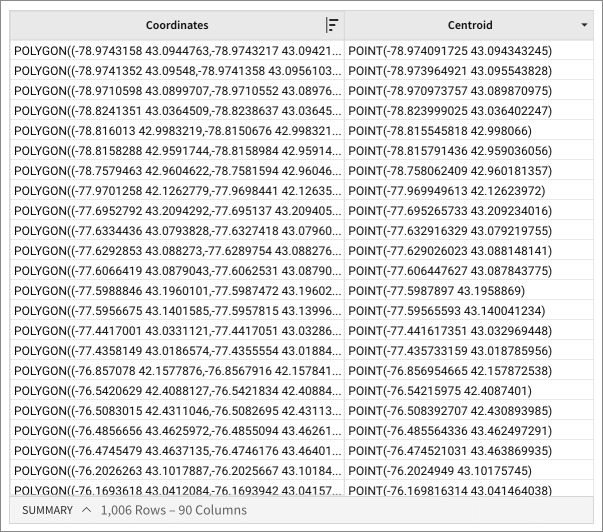# Centroid

The Centroid function calculates the geographic center of a polygon. It returns a single point.
You could use the Centroid function to determine the practical distance between two polygons.

You can use the Centroid function on Snowflake and BigQuery connections.

Centroid is one of Sigma's Geography functions.

## Syntax

`Centroid(polygon)`

The function argument is:

polygon
Required
The object for which we calculate the geometric center
Must be in valid Geography polygon format.

## Examples

`Centroid([Coordinates])`

Calculates the exact center of a polygon in column Coordinates: